Next:4.5 Splay TreesUp:4.4 Binary Search TreePrevious:4.4 Binary Search Tree

## 4.4.1 Average Case Analysis of BST Operations

RESULT

If we insert n random elements into an initially empty BST, then the average path length from the root to a node is O(log n)

• Note that the BST is formed by insertions only. Obviously the tree so formed need not be complete.
• We shall assume that all orders of n inserted elements are equally likely. This means that any of the n! permutations is equally likely to be the sequence of keys inserted.
 Let P(n) = average path length in a BST with n nodes (average number of nodes on the path from the root to a node, not necessarily a leaf) Let a = first element inserted. This will be the root of the BST. Also this is equally likely to be the first, second . . . , ith, . . . , or nth in the sorted order of the n elements.

Note that P(0) = 0 and P(1) = 1. Consider a fixed i, 0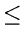in - 1. If i elements are less than a, the BST will look like in Figure 4.16.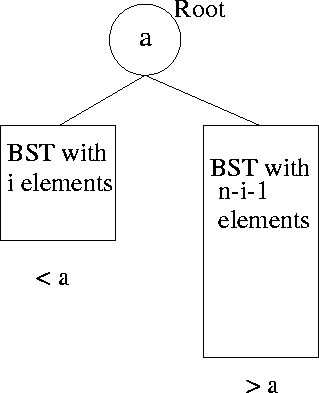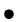Since all orders for the i small elements are equally likely and likewise for the (n - i - 1) larger elements,

Average path length in the

• left subtree = P(i)
• right subtree = P(n - i - 1)For a fixed i, let us compute the average path length of the above tree.
Number of probes if the element a is being sought = 1
Average number of probes if an element from the LST is sought = 1+P(i)
Average number of probes if an element from the RST is sought = 1 + P(n - i - 1)
Probability of seeking any of the n elements =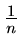$Thus, average path length for a fixed$i
 =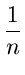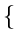1 + i(1 + P(i)) + (n - i - 1)(1 + P(n - i - 1))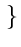= 1 +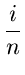P(i) +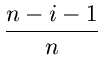P(n - i - 1) = P(n, i),   say.Observe that P(n) is given by
P(n) =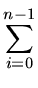Prob{LST has $i$ nodes }P(n, i)

Since the probability that the LST has i elements which is the same as the probability that a is the (i + 1th element (where i = 0, 1,..., n - 1) =, we have
 P(n) =P(n, i) =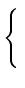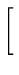1 +P(i) +P(n - i - 1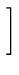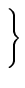= 1 +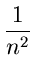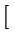iP(i) + (n - i - 1)P(n - i - 1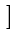= 1 +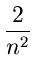iP(i)

sinceiP(i) =(n - i - 1)P(n - i - 1)Thus the average path length in a BST satisfies the recurrence:
P(n) = 1 +iP(i)We shall show that P(n1 + 4log n, by Induction.
Basis:
P(1) is known to be 1. Also the RHS = 1 for n = 1
Induction:
Let the result be true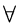i < n. We shall show that the above is true for i = n.

Consider

 P(n)1 +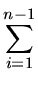i(1 + 4log i) = 1 +4ilogi +i1 +4ilogi +tex2html_image_mark>#tex2html_wrap_indisplay25297#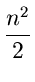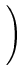sinceiThus
P(n2 +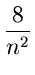ilog i
Nowilog i =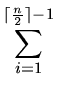ilogi +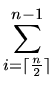ilog iilog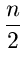+ilog n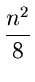log+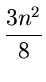log n =logn -Therefore
P(n2 +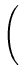log -= 1 + 4log n
A more careful analysis can be done and it can be shown that

P(n1 + 1.4log nNext:4.5 Splay TreesUp:4.4 Binary Search TreePrevious:4.4 Binary Search Tree
eEL,CSA_Dept,IISc,Bangalore